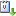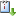Usted está aquí: A semilinear curl-curl problem in $$\mathbb{R}^3$$

# A semilinear curl-curl problem in $$\mathbb{R}^3$$

Ponente: Andrzej Szulkin
Institución: Stockholm University
Tipo de Evento: Investigación
Cuándo 23/10/2018 de 11:00 a 12:00 Salón de seminarios "Graciela Salicrup"vCaliCal

Abstract.

We look for nontrivial solutions to the system of semilinear equations

(*)   $$\nabla\times\nabla\times E = f(x,E) \text{ in } \mathbb{R}^3,$$

where  $E: \mathbb{R}^3\to\mathbb{R}^3$  and  $f=\nabla_EF$. This system arises when looking for time-periodic solutions to Maxwell equations with nonlinear polarization.

The main difficulties here are due to unboundedness of the domain and infinite dimension of the kernel of the left-hand side of (*).

We first discuss an abstract result for a class of functionals related to this problem and then apply it to prove the existence of a ground state solution as well as infinitely many geometrically distinct solutions to (*) for some classes of  $f$.

This talk is based on a work in progress with J. Mederski and J. Schino.

archivado en: